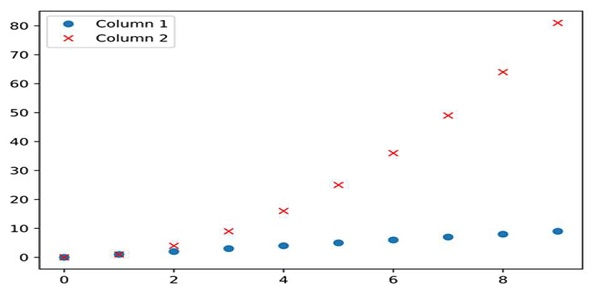# How to plot a high resolution graph in Matplotlib?

We can use the resolution value, i.e., dots per inch, and the image format to plot a high-resolution graph in Matplotlib.

## Steps

• Create a dictionary with Column 1 and Column 2 as the keys and Values are like i and i*i, where i is from 0 to 10, respectively.

• Create a data frame using pd.DataFrame(d); d created in step 1.

• Plot the data frame with ‘o’ and ‘rx’ style.

• To save the file in pdf format, use savefig() method where the image name is myImagePDF.pdf, format="pdf".

• We can set the dpi value to get a high-quality image.

• Using the saving() method, we can save the image with format=”png” and dpi=1200.

• To show the image, use the plt.show() method.

## Example

import pandas as pd
from matplotlib import pyplot as plt

d = {'Column 1': [i for i in range(10)], 'Column 2': [i * i for i in range(10)]}

df = pd.DataFrame(d)

df.plot(style=['o', 'rx'])
resolution_value = 1200
plt.savefig("myImage.png", format="png", dpi=resolution_value)
plt.show()

## Output